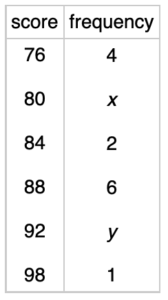# The Last Math ExamThe table above represents scores for the last math exam. If the mean of the class was exactly 87, determine possible solutions for x and y. Explain your solution.

How many solutions exist? Why?